Register for Maths, Science, English, Reasoning Olympiad Exams Register here | Book Free Demo for Live Olympiad Classes here | Check Olympiad Exam Dates here | Buy Practice Papers for IMO, IOM, HEO, IOEL etc here | Login here to participate in all India free mock test on every Saturday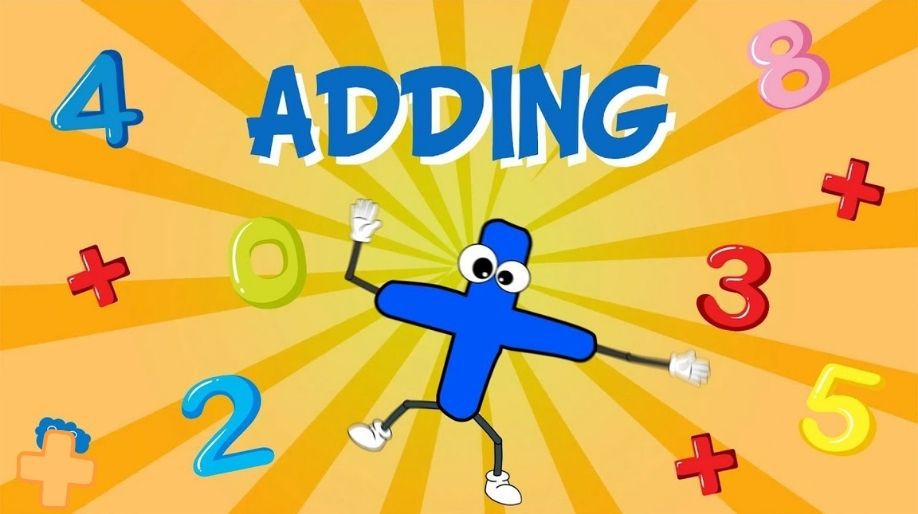What is Addition? An addition is the process of finding the total or sum by combining of two numbers, digits or values. Do you want to know what is 1+1 or 15+5? Know concept of addition and do the total of all values.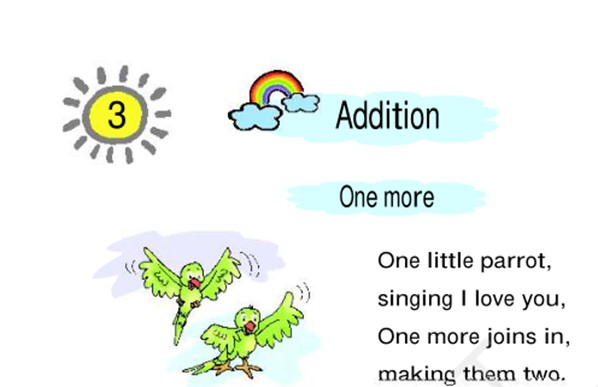Addition of two single Digit Number

For finding total of two single digit numbers, just count first number on your fingers and then count second number on your finger and sum of total fingers is the resullt.

Let’s understand with an example: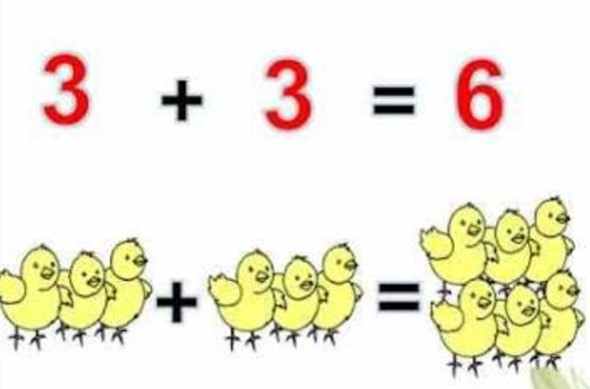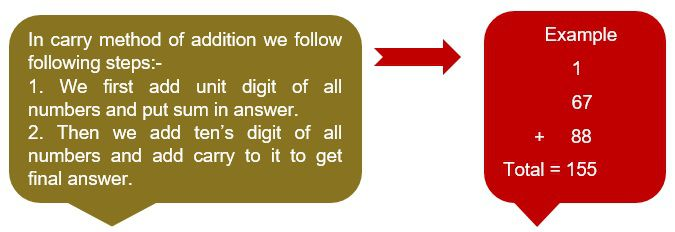Practice Questions

Practice Questions on addition of single digit number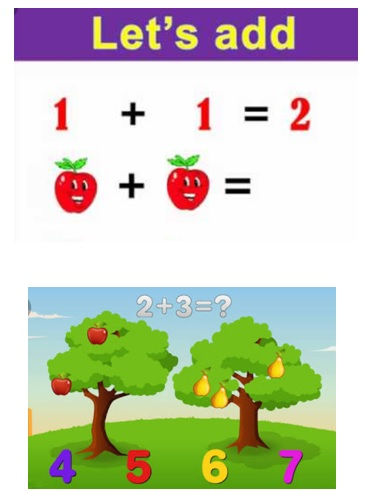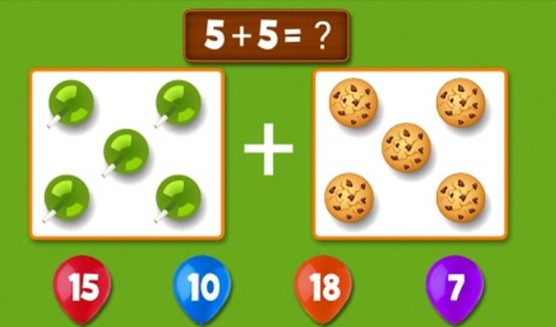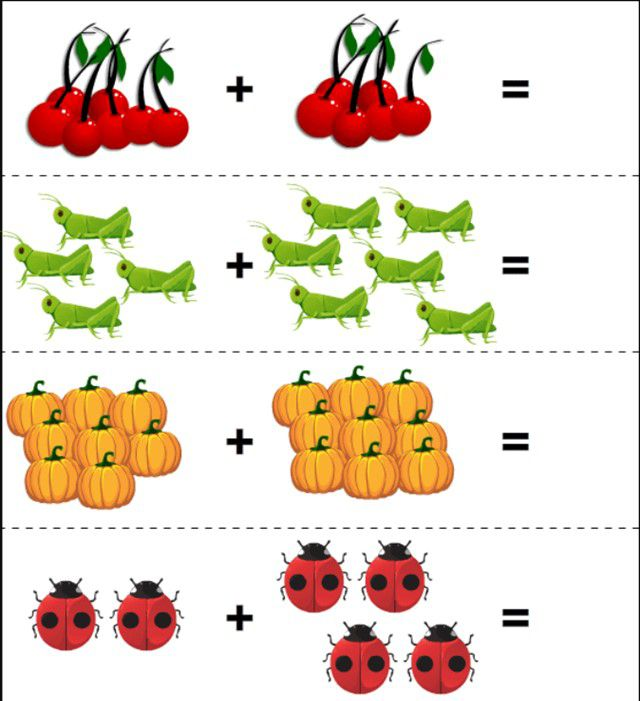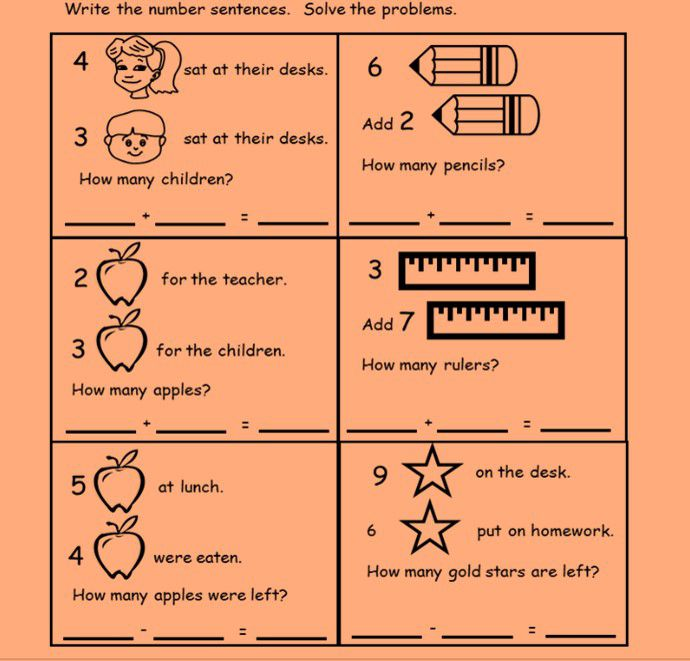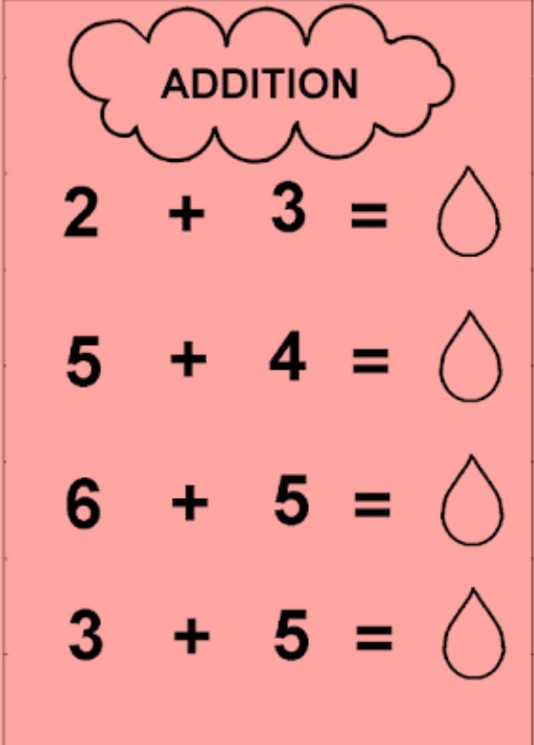Practice Questions by using Carry Method

Example of addition of single digit number with two digit number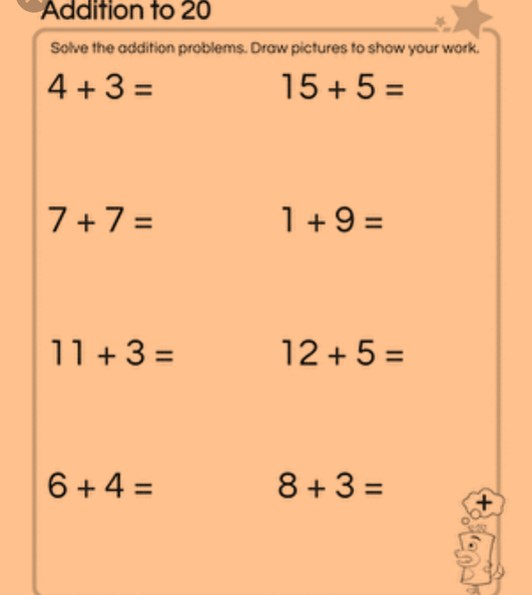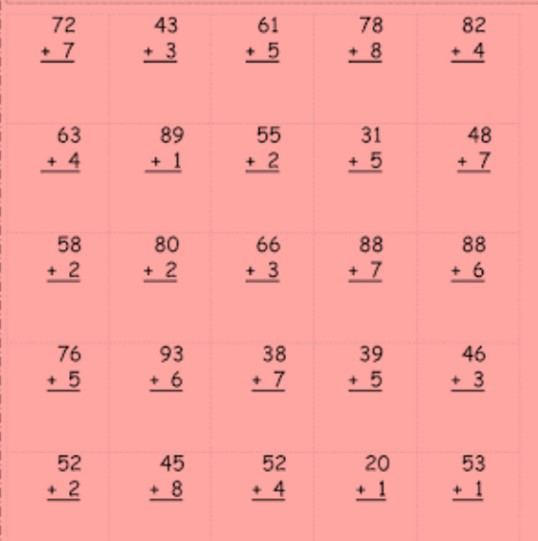Practice Questions on Addition of two digit numbers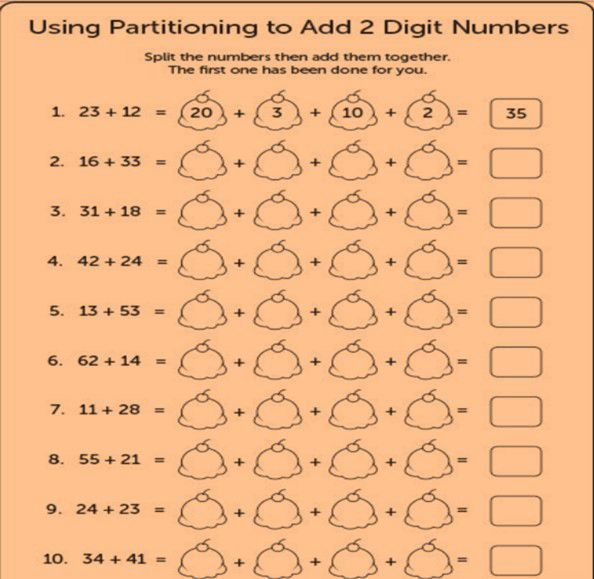Practice questions on addition of three digit numbers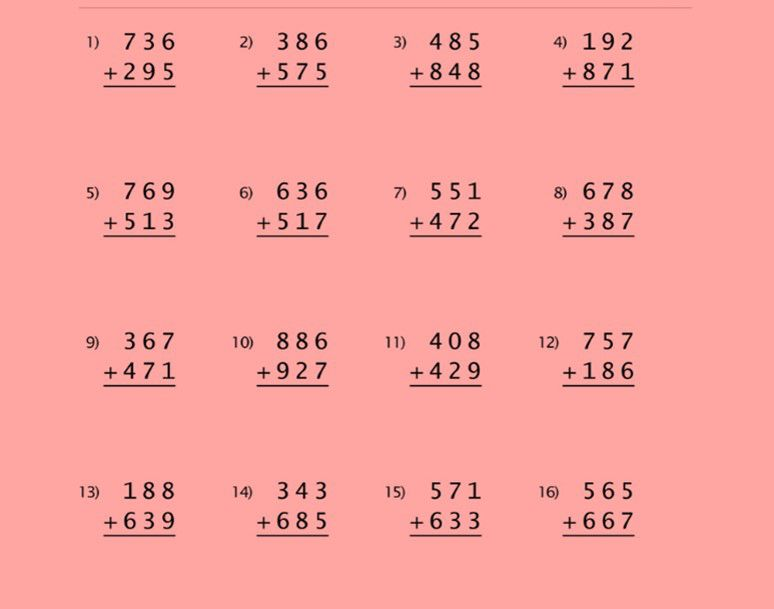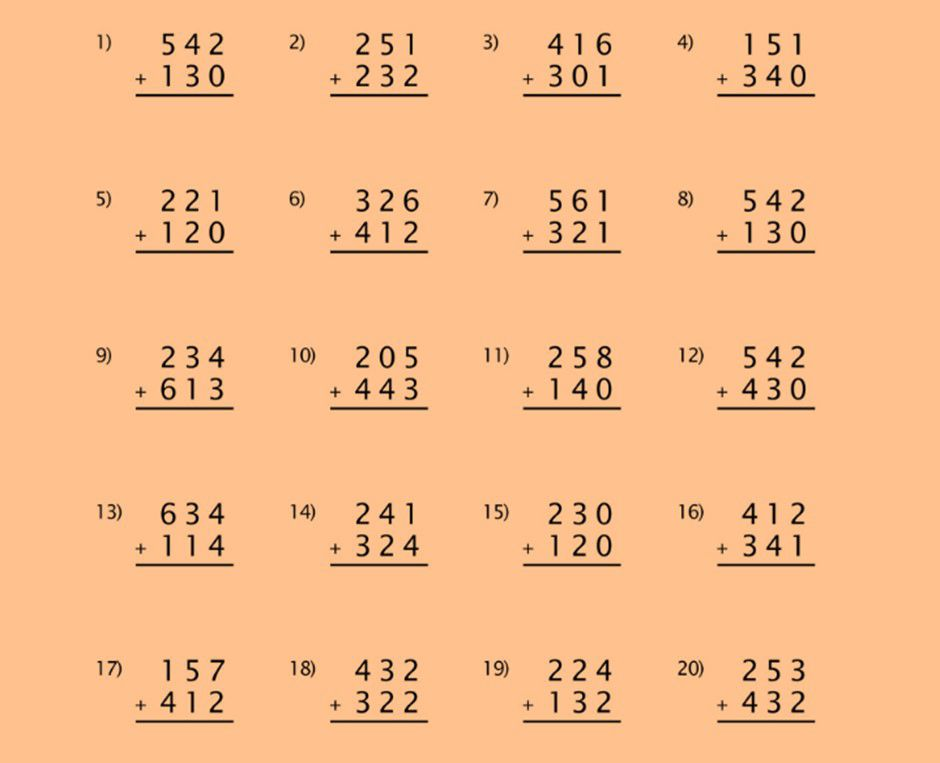Recap

• An addition is a process of finding the total or sum by combining of two numbers, digits or values.
• Addition of two single digit numbers can be done by using fingers of the hand.
• Carry method is used to add two numbers with more than 1 digit.

 Q.1 Add the numbers: 4+3=?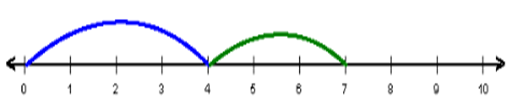a) 3 b) 7 c) 4 d) 2
 Q.2 Add the numbers:a) 1 b) 4 c) 5 d) 3
 Q.3 Count, add the pictures and choose the correct option: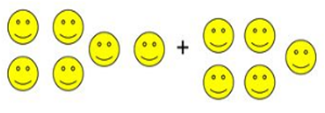a) 6 b) 5 c) 11 d) 10
 Q.4 Count, add the pictures and choose the correct option: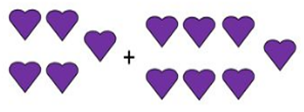a) 7 b) 12 c) 5 d) 2
 Q.5 Count, add the pictures and choose the correct option: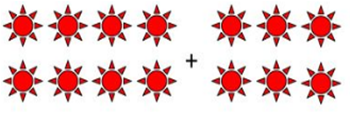a) 6 b) 2 c) 8 d) 14
 Q.6 Count, add the pictures and choose the correct option:a) 15 b) 14 c) 13 d) 12
 Q.7 Count, add the pictures and choose the correct option:a) 4 b) 3 c) 7 d) 6
 Q.8 Count, add the pictures and choose the correct option: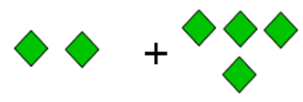a) 2 b) 6 c) 3 d) 7
 Q.9 Count, add the pictures and choose the correct option: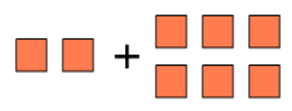a) 8 b) 10 c) 6 d) 2
 Q.10 Add the numbers: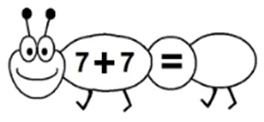a) 14 b) 13 c) 12 d) 11